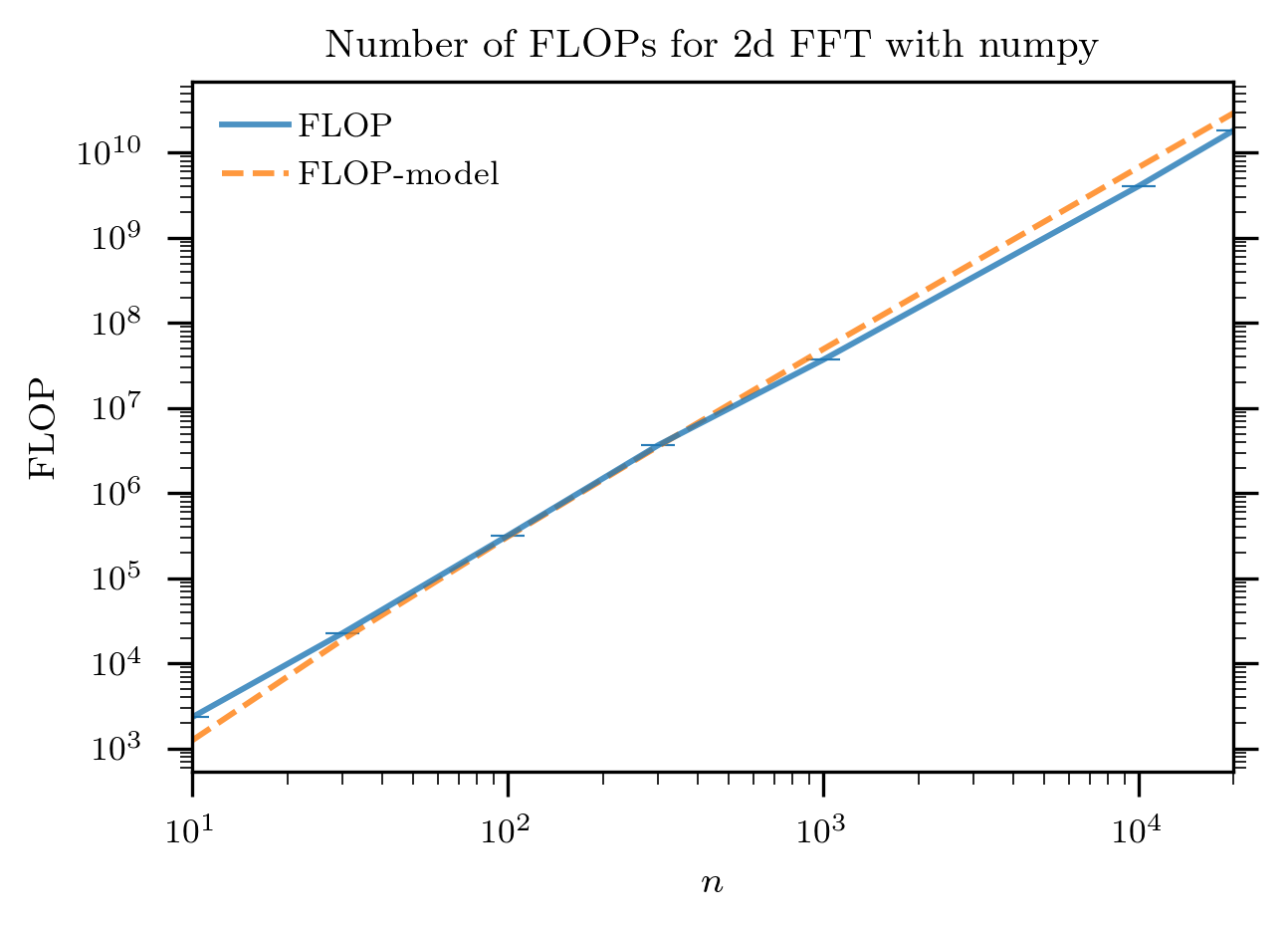On the Linux command line it is fairly easy to use the `perf` command to measure number of floating point operations (or other performance metrics). (See for example this old blog post ) with this approach it is not easy to get a fine grained view of how different stages of processings within a single process. In this short note I describe how the `python-papi` package can be used to measure the FLOP requirements of any section of a Python program.

## Installation

The package is available from pypi so you can install it in the usual way:

``````pipenv install python-papi
``````

If you don’t use `pipenv`, replace with your normal Python package management scheme.

If you are not running root (which of course you should not be), it is necessary to ensure the kernel paranoid switch is set appropriately:

``````sudo sh -c 'echo 1 >/proc/sys/kernel/perf_event_paranoid'
``````

Note that the floating point counters are available on many recent Intel processors, however they are not supported on Haswell architecture (e..g, the v3 Xeon processors). They work on Ivy & Sandy Bridge and Broadwell and subsequent architectures.

Note If you get a `PAPI_ENOEVNT` error then most likely the CPU you are using does not support the right counters. In this case you will need to do your testing on another CPU. In case you are counting Floating Point Operations, this count should depend on the algorithm itself rather than the CPU, so a measurement on any CPU is sufficient for understanding the algorithm.

## Usage

The counters can be used very easily, e.g.:

``````from pypapi import events, papi_high as high

high.start_counters([events.PAPI_FP_OPS,])
# Do something
x=high.stop_counters()
``````

and the number of floating point operations will be in the variable `x`. Fuller documentation for PAPI is available at here.

## Example

As a simple example, I count here the number of floating point operations used to compute `numpy.fft` on a two-dimensional array.

The measurement code is as follows:

``````for n in [10, 30, 100, 300, 1000, 10000, 20000]:
aa=numpy.mgrid[0:n:1,0:n:1]
high.start_counters([events.PAPI_FP_OPS,])
a=numpy.fft.fft(aa)
x=high.stop_counters()
print (n, x) # Or record results in another way
``````

The results are plotted below:together with the model: `4*numpy.log(n**2)*n**2-6*n**2 + 8`. It can be seen that this model is fairly close to the number of operations as captured by the CPU performance monitoring unit.

Other Examples See for example the post applying this to PyTorch and insight that can be derived and a roofline-like model